# 一、决策树原理

J.R.Quinlan的工作主要是引进了信息论中的信息增益，他将其称为信息增益（information gain），作为属性判别能力的度量，设计了构造决策树的递归算法。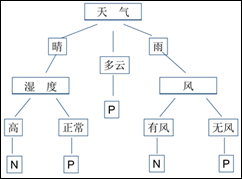ID3就是要从表的训练集构造图这样的决策树。实际上，能正确分类训练集的决策树不止一棵。Quinlan的ID3算法能得出结点最少的决策树。

ID3算法：

⒈ 对当前例子集合，计算各属性的信息增益；

⒉ 选择信息增益最大的属性Ak

⒊ 把在Ak处取值相同的例子归于同一子集，Ak取几个值就得几个子集；

⒋ 对既含正例又含反例的子集，递归调用建树算法；

⒌ 若子集仅含正例或反例，对应分枝标上P或N，返回调用处。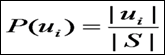|S|表示例子集S的总数，|ui|表示类别ui的例子数。对9个正例和5个反例有：

P（u1）=9/14

P（u2）=5/14

H（S）=（9/14）log（14/9）+（5/14）log（14/5）=0.94bit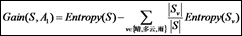S=[9+,5-] //原样例集中共有14个样例，9个正例，5个反例

S晴=[2+,3-]//属性A1取值晴的样例共5个，2正，3反

S多云=[4+,0-] //属性A1取值多云的样例共4个，4正，0反

S雨=[3+,2-] //属性A1取值晴的样例共5个，3正，2反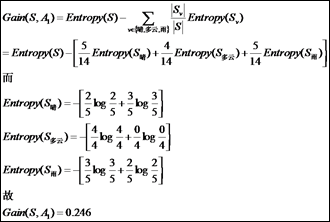3.结果为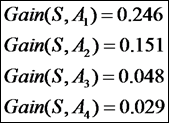4.建决策树的根和叶子

ID3算法将选择信息增益最大的属性天气作为树根，在14个例子中对天气的3个取值进行分枝，3 个分枝对应3 个子集，分别是: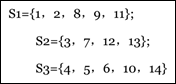5.递归建树

（1）对S1，湿度属性信息增益最大，以它为该分枝的根结点，再向下分枝。湿度取高的例子全为N类，该分枝标记N。取值正常的例子全为P类，该分枝标记P。

（2）对S3，风属性信息增益最大，则以它为该分枝根结点。再向下分枝，风取有风时全为N类，该分枝标记N。取无风时全为P类，该分枝标记P。

# 二、PYTHON实现决策树算法分类

1、计算给定数据shangnon数据的函数：

[python] view plaincopy

1. def calcShannonEnt(dataSet):

2. #calculate the shannon value

3. numEntries = len(dataSet)

4. labelCounts = {}

5. for featVec in dataSet: #create the dictionary for all of the data

6. currentLabel = featVec[-1]

7. if currentLabel not in labelCounts.keys():

8. labelCounts[currentLabel] = 0

9. labelCounts[currentLabel] += 1

10. shannonEnt = 0.0

11. for key in labelCounts:

12. prob = float(labelCounts[key])/numEntries

13. shannonEnt -= prob*log(prob,2) #get the log value

14. return shannonEnt

2. 创建数据的函数

[python] view plaincopy

1. def createDataSet():

2. dataSet = [[1,1,'yes'],

3. [1,1, 'yes'],

4. [1,0,'no'],

5. [0,1,'no'],

6. [0,1,'no']]

7. labels = ['no surfacing','flippers']

8. return dataSet, labels

3.划分数据集，按照给定的特征划分数据集

[python] view plaincopy

1. def splitDataSet(dataSet, axis, value):

2. retDataSet = []

3. for featVec in dataSet:

4. if featVec[axis] == value: #abstract the fature

5. reducedFeatVec = featVec[:axis]

6. reducedFeatVec.extend(featVec[axis+1:])

7. retDataSet.append(reducedFeatVec)

8. return retDataSet

4.选择最好的数据集划分方式

[python] view plaincopy

1. def chooseBestFeatureToSplit(dataSet):

2. numFeatures = len(dataSet)-1

3. baseEntropy = calcShannonEnt(dataSet)

4. bestInfoGain = 0.0; bestFeature = -1

5. for i in range(numFeatures):

6. featList = [example[i] for example in dataSet]

7. uniqueVals = set(featList)

8. newEntropy = 0.0

9. for value in uniqueVals:

10. subDataSet = splitDataSet(dataSet, i , value)

11. prob = len(subDataSet)/float(len(dataSet))

12. newEntropy +=prob * calcShannonEnt(subDataSet)

13. infoGain = baseEntropy - newEntropy

14. if(infoGain > bestInfoGain):

15. bestInfoGain = infoGain

16. bestFeature = i

17. return bestFeature

5.递归创建树

[python] view plaincopy

1. def majorityCnt(classList):

2. classCount = {}

3. for vote in classList:

4. if vote not in classCount.keys(): classCount[vote] = 0

5. classCount[vote] += 1

6. sortedClassCount = sorted(classCount.iteritems(), key=operator.itemgetter(1), reverse=True)

7. return sortedClassCount

[python] view plaincopy

1. def createTree(dataSet, labels):

2. classList = [example[-1] for example in dataSet]

3. # the type is the same, so stop classify

4. if classList.count(classList) == len(classList):

5. return classList

6. # traversal all the features and choose the most frequent feature

7. if (len(dataSet) == 1):

8. return majorityCnt(classList)

9. bestFeat = chooseBestFeatureToSplit(dataSet)

10. bestFeatLabel = labels[bestFeat]

11. myTree = {bestFeatLabel:{}}

12. del(labels[bestFeat])

13. #get the list which attain the whole properties

14. featValues = [example[bestFeat] for example in dataSet]

15. uniqueVals = set(featValues)

16. for value in uniqueVals:

17. subLabels = labels[:]

18. myTree[bestFeatLabel][value] = createTree(splitDataSet(dataSet, bestFeat, value), subLabels)

19. return myTree

[python] view plaincopy

1. myDat, labels = trees.createDataSet()

2. myTree = trees.createTree(myDat,labels)

3. print myTree

{'no surfacing': {0: 'no', 1: {'flippers': {0: 'no', 1: 'yes'}}}}

6.实用决策树进行分类的函数

[python] view plaincop

1. def classify(inputTree, featLabels, testVec):

2. firstStr = inputTree.keys()

3. secondDict = inputTree[firstStr]

4. featIndex = featLabels.index(firstStr)

5. for key in secondDict.keys():

6. if testVec[featIndex] == key:

7. if type(secondDict[key]).__name__ == 'dict':

8. classLabel = classify(secondDict[key], featLabels, testVec)

9. else: classLabel = secondDict[key]

10. return classLabel

[python] view plaincopy

1. trees.classify(myTree,labels,[1,0])

'no'

Congratulation. Oh yeah. You did it.!!!

## 1、决策树算法

J. Ross Quinlan在1975提出将信息熵的概念引入决策树的构建，这就是鼎鼎大名的ID3算法。后续的C4.5, C5.0, CART等都是该方法的改进。

[plain] view plaincopy

1. 样本 红 大 好苹果

2. 0 1 1 1

3. 1 1 0 1

4. 2 0 1 0

5. 3 0 0 0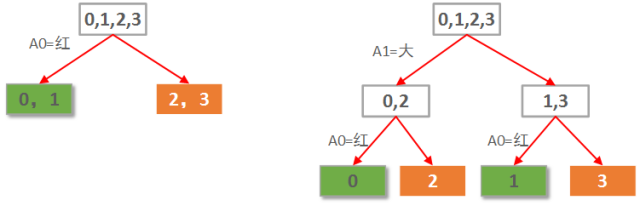0，1叶子节点有2个正例，0个负例。信息熵为：e1 = -(2/2 * log(2/2) + 0/2 * log(0/2)) = 0。

2，3叶子节点有0个正例，2个负例。信息熵为：e2 = -(0/2 * log(0/2) + 2/2 * log(2/2)) = 0。

0，2子节点有1个正例，1个负例。信息熵为：e1 = -(1/2 * log(1/2) + 1/2 * log(1/2)) = 1。

1，3子节点有1个正例，1个负例。信息熵为：e2 = -(1/2 * log(1/2) + 1/2 * log(1/2)) = 1。

## 2、数据集

[plain] view plaincopy

1. 1.5 50 thin

2. 1.5 60 fat

3. 1.6 40 thin

4. 1.6 60 fat

5. 1.7 60 thin

6. 1.7 80 fat

7. 1.8 60 thin

8. 1.8 90 fat

9. 1.9 70 thin

10. 1.9 80 fat

（数据是作者主观臆断，具有一定逻辑性，但请无视其合理性）

## 3、Python实现

Python代码实现如下：

[python] view plaincopy

1. # -*- coding: utf-8 -*-

2. import numpy as np

3. import scipy as sp

4. from sklearn import tree

5. from sklearn.metrics import precision_recall_curve

6. from sklearn.metrics import classification_report

7. from sklearn.cross_validation import train_test_split

8.

9.

10. ''''' 数据读入 '''

11. data = []

12. labels = []

13. with open("data\\1.txt") as ifile:

14. for line in ifile:

15. tokens = line.strip().split(' ')

16. data.append([float(tk) for tk in tokens[:-1]])

17. labels.append(tokens[-1])

18. x = np.array(data)

19. labels = np.array(labels)

20. y = np.zeros(labels.shape)

21.

22.

23. ''''' 标签转换为0/1 '''

24. y[labels=='fat']=1

25.

26. ''''' 拆分训练数据与测试数据 '''

27. x_train, x_test, y_train, y_test = train_test_split(x, y, test_size = 0.2)

28.

29. ''''' 使用信息熵作为划分标准，对决策树进行训练 '''

30. clf = tree.DecisionTreeClassifier(criterion='entropy')

31. print(clf)

32. clf.fit(x_train, y_train)

33.

34. ''''' 把决策树结构写入文件 '''

35. with open("tree.dot", 'w') as f:

36. f = tree.export_graphviz(clf, out_file=f)

37.

38. ''''' 系数反映每个特征的影响力。越大表示该特征在分类中起到的作用越大 '''

39. print(clf.feature_importances_)

40.

41. '''''测试结果的打印'''

43. print(x_train)

45. print(y_train)

47.

48. '''''准确率与召回率'''

49. precision, recall, thresholds = precision_recall_curve(y_train, clf.predict(x_train))

51. print(classification_report(y, answer, target_names = ['thin', 'fat']))

[ 0.2488562 0.7511438]
array([[ 1.6, 60. ],
[ 1.7, 60. ],
[ 1.9, 80. ],
[ 1.5, 50. ],
[ 1.6, 40. ],
[ 1.7, 80. ],
[ 1.8, 90. ],
[ 1.5, 60. ]])
array([ 1., 0., 1., 0., 0., 1., 1., 1.])
array([ 1., 0., 1., 0., 0., 1., 1., 1.])
1.0

precision recall f1-score support
thin 0.83 1.00 0.91 5
fat 1.00 0.80 0.89 5

avg / total 1.00 1.00 1.00 8

array([ 0., 1., 0., 1., 0., 1., 0., 1., 0., 0.])
array([ 0., 1., 0., 1., 0., 1., 0., 1., 0., 1.])

1、拆分训练数据与测试数据

2、特征的不同影响因子。

3、准确率与召回率

[plain] view plaincopy

1. digraph Tree {

2. 0 [label="X <= 55.0000\nentropy = 0.954434002925\nsamples = 8", shape="box"] ;

3. 1 [label="entropy = 0.0000\nsamples = 2\nvalue = [ 2. 0.]", shape="box"] ;

4. 0 -> 1 ;

5. 2 [label="X <= 70.0000\nentropy = 0.650022421648\nsamples = 6", shape="box"] ;

6. 0 -> 2 ;

7. 3 [label="X <= 1.6500\nentropy = 0.918295834054\nsamples = 3", shape="box"] ;

8. 2 -> 3 ;

9. 4 [label="entropy = 0.0000\nsamples = 2\nvalue = [ 0. 2.]", shape="box"] ;

10. 3 -> 4 ;

11. 5 [label="entropy = 0.0000\nsamples = 1\nvalue = [ 1. 0.]", shape="box"] ;

12. 3 -> 5 ;

13. 6 [label="entropy = 0.0000\nsamples = 3\nvalue = [ 0. 3.]", shape="box"] ;

14. 2 -> 6 ;

15. }

posted @ 2018-08-14 15:04  py小蟒蛇  阅读(142)  评论(0编辑  收藏